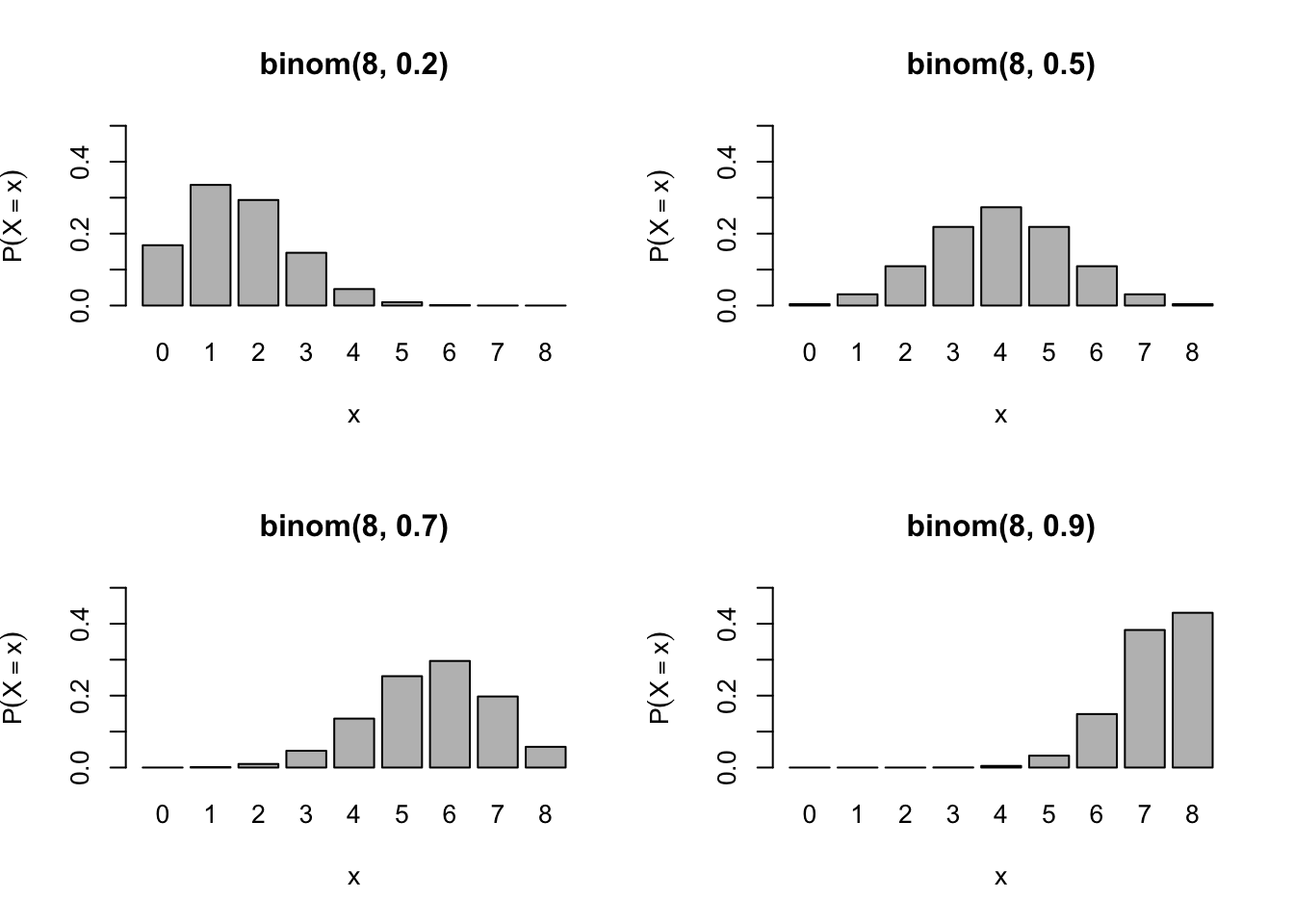These notes are based on Chapter 15 & 16 of Jones, O., Maillardet, R., & Robinson, A. (2009). Introduction to scientific programming and simulation using R. CRC Press.

Building upon Probability Theory notes, we are going to study specific probability distributions in these notes. They are so common and/or important that each has got a name and dedicated R build-in functions. Although at some point we may need to create special distributions and write associated functions for our models, it is often sufficient to combine the existing ones as building blocks. So, let’s master them and get prepared for stochastic modelling.

# Discrete distributions

R recognises common distributions by short names and specifies them by parameters.

Distribution R name R Parameters Abbreviation
Bernoulli binom size=1, prob $$\mathrm{Bernoulli}(p)$$
Binomial binom size, prob $$\mathrm{binom}(n,p)$$
Geometric geom prob $$\mathrm{geom}(p)$$
Negative binomial nbinom size, prob $$\mathrm{nbinom}(r,p)$$
Poisson pois lambda $$\mathrm{pois}(\lambda)$$

As you see below, $$\mathrm{Bernoulli}(p)$$ is equivalent to $$\mathrm{binom}(1,p)$$.

R built-in functions for common distributions follow a certain pattern. Suppose that a distribution is recognised by the name xyz in R and specified by parameters p1 and p2, then R provides the following four functions to help you work with the distribution.

• dxyz(x, p1, p2, ...) returns $$P(X=x)$$, the value of PMF at $$x$$.
• pxyz(q, p1, p2, ...) returns $$F(q)$$, the value of CDF at $$q$$.
• qxyz(p, p1, p2, ...) returns $$\arg\min_x F(x)\geq p$$, roughly equal to the value at the $$p$$-th percentile.
• rxyz(n, p1, p2, ...) returns $$n$$ random samples.

In these notes, I avoid using $$p(x)$$ for PMF but use $$P(X=x)$$ to describe the distribution of $$X$$ since we are going to use lots of $$p$$ below.

## Bernoulli

The support is $$\{0,1\}$$, which is often interpreted as “failure” and “success” respectively. Naturally, it has a single “success” parameter $$p\in(0,1)$$ that specifies the probability of “success”, i.e. $$p = P(X=1)$$.

It serves as a fundamental building block and, as you will see below, many complex distributions can be modelled as a sequence of independent Bernoulli trials.

\begin{align} P(X=x) &= \begin{cases} p & \text{for } x=1\\ 1 - p & \text{for } x=0 \end{cases}\\\\ \mathbb{E}X &= \sum_{x\in\{0,1\}} xP(X=x)\\ &= 0\cdot(1-p)+1\cdot p\\ &= p\\\\ \operatorname{Var} X &= \sum_{x\in\{0,1\}} (x-p)^2P(X=x)\\ &= (0-p)^2\cdot(1-p) + (1-p)^2\cdot p\\ &= p(1-p) \end{align}

The support of $$X$$ means the set $$\{x\in\mathbb{R} : P(X=x)>0\}$$ for discrete $$X$$ or $$\{x\in\mathbb{R} : f(x)>0\}$$ for continuous $$X$$ where $$f$$ is the PDF of $$X$$.

## Binomial

Imagine doing independent Bernoulli($$p$$) trials $$n$$ times. Let $$X$$ denote the number of $$1$$ out of $$n$$ trials. Then, $$X\sim \text{binom}(n,p)$$.

Formally, let $$B_1,\dots,B_n \sim \text{Bernoulli}(p)$$. Then, $X = B_1+\dots+B_n .$ Given the $$n$$ Bernoulli trials, the support is $$\{0,1,\dots,n\}$$.

In deriving the PMF, a key computation is to count the number of ways to choose $$x$$ trials that turn out $$1$$ from the total $$n$$ trials, commonly denoted by $$\binom{n}{x}$$.

\begin{align} P(X=x) &= \binom{n}{x}p^x(1-p)^{n-x}\\\\ \mathbb{E}X &= \mathbb{E}(B_1+\dots+B_n)\\ &= \mathbb{E}B_1+\dots+\mathbb{E}B_n\\ &= np\\\\ \operatorname{Var} X &= \operatorname{Var}(B_1+\dots+B_n)\\ &= \operatorname{Var}B_1+\dots+\operatorname{Var}B_n\\ &= np(1-p) \end{align}

$$\operatorname{Var} X$$ formula is drived by exploiting $$n$$ independent Bernoulli trials.

Here are some of PMF plots with varying $$p$$.

n <- 8
x <- 0:n
myplot <- function(p) {
barplot(dbinom(x,n,p),
names.arg = x,
ylim=c(0,.5),
main=paste("binom(",n,", ",p,")", sep=""),
xlab="x",
ylab=expression(P(X==x)))
}
par(mfrow=c(2,2))
for (p in c(.2,.5,.7,.9)) {
myplot(p)
}## Poisson

Imagine a large number of independent Bernoulli trials, in each of which success is very rare. For example, the number of car accidents in Melbourne in a single day, where each of thousands of drivers has a very small chance of accident.

While we can use binom($$n,p$$) with large $$n$$ and small $$p$$, we could be in trouble because computers cannot handle extremely large or small values very well. An alternative modelling option is pois($$\lambda$$) with $$\lambda\approx np$$. Here is why.

Let $$Y_n \sim \text{binom}(n,p)$$ such that $$p=\lambda/n$$ for some $$\lambda>0$$. Then,

\begin{align} P(Y_n=x) &= \binom{n}{x}p^x(1-p)^{n-x}\\ &= \frac{n\cdot(n-1)\dots(n-x+1)}{x!}\left(\frac{\lambda}{n}\right)^x\left(1-\frac{\lambda}{n}\right)^{n-x}\\ &= 1\cdot\frac{n-1}{n}\dots\frac{n-x+1}{n}\frac{\lambda^x}{x!}\left(1-\frac{\lambda}{n}\right)^{n}\left(1-\frac{\lambda}{n}\right)^{-x}\\ &\to 1\cdot 1\dots 1\frac{\lambda^x}{x!}e^{-\lambda}\cdot 1 \text{ as } n\to\infty\\ &= \frac{e^{-\lambda}\lambda^x}{x!} \end{align}

which is the PMF of pois($$\lambda$$). The limit $\lim_{n\to\infty} P(Y_n=x) = \frac{e^{-\lambda}\lambda^x}{x!}$ is called the law of rare events.

As indicated by $$n\to\infty$$, the support is $$\mathbb{Z}_{\geq0}=\{0,1,\dots\}$$.

Both mean and variance are equal to $$\lambda$$.

An important property of $$\text{pois}(\lambda)$$ is the following. If $$X_1,\dots,X_n$$ is an IID sequence of $$\text{pois}(\lambda)$$, then $X_1+\dots+X_n = \text{pois}(\lambda_1+\dots+\lambda_n) .$ Here is a proof for $$n=2$$, which lets us generalise to $$n>2$$ by induction.

\begin{align} P(X_1+X_2=n) &= \sum_{m=0}^n P(X_1=m)P(X_2=n-m)\\ &= \sum_{m=0}^n \frac{e^{-\lambda_1} \lambda_1^m}{m!}\cdot\frac{e^{-\lambda_2} \lambda_2^{n-m}}{(n-m)!}\\ &= \frac{e^{-(\lambda_1+\lambda_2)} (\lambda_1+\lambda_2)^n}{n!} \cdot \sum_{m=0}^n\binom{n}{m}\left(\frac{\lambda_1}{\lambda_1+\lambda_2}\right)^m\left(\frac{\lambda_2}{\lambda_1+\lambda_2}\right)^{n-m}\\ &= \frac{e^{-(\lambda_1+\lambda_2)} (\lambda_1+\lambda_2)^n}{n!} \end{align}

because the summand is the PMF for $$\text{binom}\left(n,\frac{\lambda_1}{\lambda_1+\lambda_2}\right)$$.

Let’s compare $$X\sim \text{pois}(\lambda)$$ and $$Y\sim \text{binom}(n,p)$$ such that $$p=\lambda/n$$ with large $$n$$. Suppose $$\lambda=1$$ for simplicity and $$n=10000$$.

lambda <- 1
n <- 10000
x <- c(0,2,5)
$$x$$ $$P(X=x)$$ $$P(Y=x)$$
0 0.3678794 0.367861
2 0.1839397 0.1839489
5 0.0030657 0.003064

Here are some of PMF plots with varying $$\lambda$$.

n <- 12
x <- 0:n
myplot <- function(l) {
barplot(dpois(x,l),
names.arg = x,
ylim=c(0,.6),
main=paste("pois(",l,")", sep=""),
xlab="x",
ylab=expression(P(X==x)))
}
par(mfrow=c(2,2))
for (l in c(.5,1,2,5)) {
myplot(l)
}